Auxiliary Materials for Perfect Parallelepipeds Exist

by Jorge Sawyer and Cliff Reiter

In:=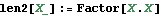In:=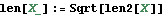In:=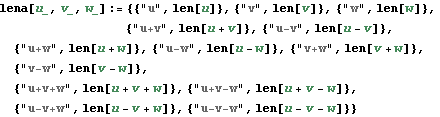In:=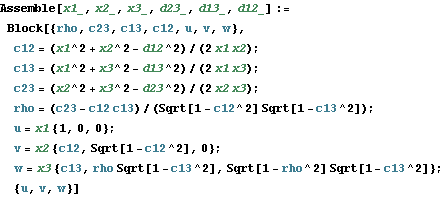Verification of Theorem 1

In:=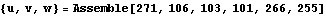Out=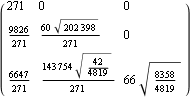In:=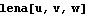Out=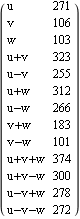Verification of Theorem 4

In:=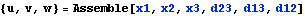Out=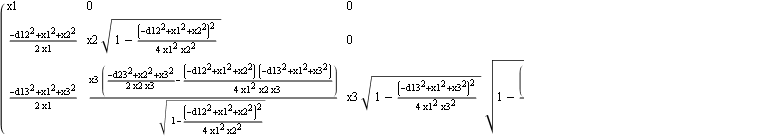In:=Out=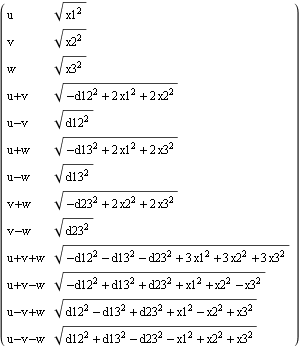Created by Wolfram Mathematica 6.0  (30 June 2009)